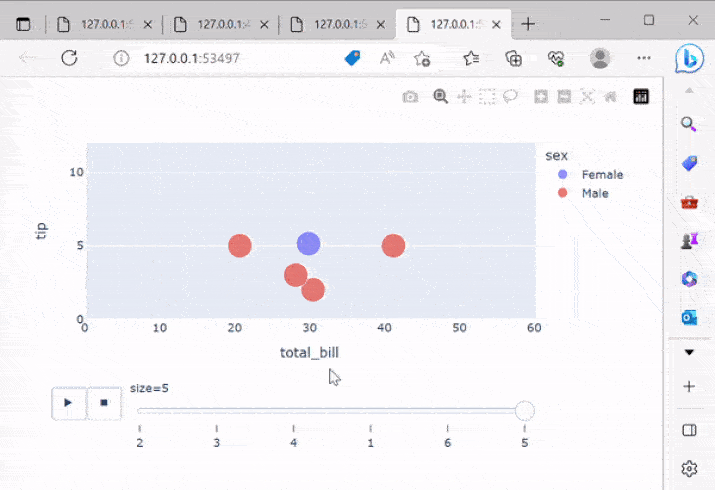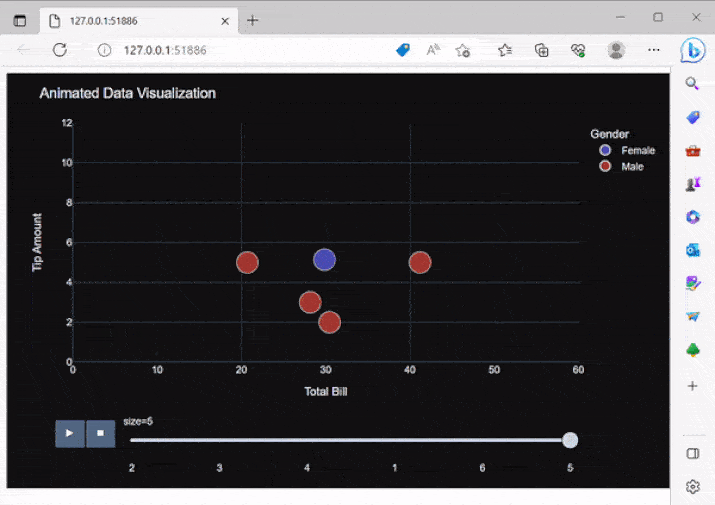# Animated Data Visualization using Plotly Express

Animated data visualization is now an essential tool for data analysis, as it provides a clear and dynamic way to explore trends and patterns over time, this can be done with the help of a Python library known as Plotly Express, which is used to create these visualizations easily and intuitively and also provides a high-level interface for creating interactive plots.

In this article, we will be discussing how to perform Animated data visualization using Plotly Express.

## The Power of Animation in Data Visualization

Animated data visualization takes storytelling with data to a whole new level. By adding motion and time-based transitions to visualizations, we can uncover patterns, trends, and relationships that might be missed in static representations. Animation helps to engage viewers, guide their focus, and enhance their understanding of complex datasets.

### Plotly Express

Plotly Express, built on top of the Plotly library, offers an intuitive and streamlined interface for creating animated data visualizations. With its extensive documentation and a vast collection of inbuilt datasets, Plotly Express makes it easy to prototype and experiment with different visualizations.

## Animated data visualization using Plotly Express

Creating an animated data visualization using Plotly Express is a simple and straightforward process.

### Preparing the Data

Before diving into creating animated visualizations, it is essential to have the data ready. Plotly Express supports various data formats, including CSV, Excel, and Pandas DataFrames. Once the data is loaded, it can be cleaned, transformed, and filtered to extract relevant insights.

### Creating Animated Visualizations

Plotly Express provides a wide range of animated plot types, allowing users to choose the most suitable visualization for their data. Let's take a look at the steps to create an animated scatter plot using Plotly Express −

• Step 1 − Install Plotly and Plotly Express: Begin by installing the necessary libraries. You can use pip to install Plotly and Plotly Express.

• Step 2 − Import the required libraries: In your Python script or Jupyter Notebook, import the necessary libraries like pandas and plotly.express.

• Step 3 − Load your data: Read your data into a pandas DataFrame. Make sure your data has a column representing the values that will be visualized over time.

• Step 4 − Create an animated plot: Use the scatter function from Plotly Express to create an animated scatter plot. Specify the time-based column using the animation_frame parameter.

• Step 5 − Customize the plot (optional): You can customize various aspects of the plot, such as the title, axis labels, colors, and markers. Use the update_layout method to modify the layout and the update_traces method to customize the individual traces.

• Step 6 − Show the plot: Finally, use the show method to display the animated plot.

Below is the program for animated data visualization on the tips dataset using plotly express.

### Example

import plotly.express as px

# Step 1: Install Plotly and Plotly Express (if not already installed)
# Step 2: Import the required libraries
# Step 3: Load the inbuilt dataset
df = px.data.tips()

# Step 4: Create an animated plot
fig = px.scatter(df, x='total_bill', y='tip', animation_frame='size',
size='size', color='sex', hover_name='day',
log_x=False, log_y=False, range_x=[0, 60], range_y=[0, 12])

fig.update_traces(marker=dict(line=dict(width=1, color='white')))

# Step 6: Show the plot
fig.show()


### Output## Customization and Interaction

One of the significant advantages of Plotly Express is its flexibility in customizing visualizations. Users can adjust various elements, including titles, axes labels, colors, fonts, and templates, to match their desired style and branding. Moreover, viewers can interact with the animated visualizations, zooming in, panning, and exploring the data in real time.

### Example

import plotly.express as px

# Step 1: Install Plotly and Plotly Express (if not already installed)
# Step 2: Import the required libraries
# Step 3: Load the inbuilt dataset
df = px.data.tips()

# Step 4: Create an animated plot
fig = px.scatter(df, x='total_bill', y='tip', animation_frame='size',
size='size', color='sex', hover_name='day',
log_x=False, log_y=False, range_x=[0, 60], range_y=[0, 12])

# Step 5: Customize the plot (optional)
fig.update_layout(title='Animated Data Visualization', xaxis_title='Total Bill',
yaxis_title='Tip Amount', legend_title='Gender',
font=dict(family='Arial', size=12),
width=800, height=500,
hoverlabel=dict(font_family='Arial', font_size=12),
template='plotly_dark')
fig.update_traces(marker=dict(line=dict(width=1, color='white')))

# Step 6: Show the plot
fig.show()


### Output## Conclusion

In conclusion, Plotly Express offers a user-friendly and feature-rich platform for creating captivating animated data visualizations. By leveraging motion and transitions, these visualizations uncover hidden patterns and relationships, enhancing our understanding of complex datasets. The ability to customize various elements allows for personalized and visually appealing presentations. Whether analyzing population dynamics, tracking economic trends, or visualizing scientific experiments, animated data visualizations with Plotly.

Through the sample programs and discussions in this article, we have seen how Plotly Express allows us to create captivating animated visualizations with just a few lines of code.

Updated on: 24-Jul-2023

78 Views